# Statistical Process Control for True Position

### True Position SPC is a Two-Fold Issue

Applying SPC to true position involves two issues: 1) the control aspect and 2) capability ratios. ProFicient manages both.

True Position Control Charts

The control portion lies in the independent planes of x and y. The polar coordinate is the result of the two, but not what is controlled. Therefore, the logical place to set up control charts is one for the x values and one for the y values. Optionally, a control chart can be placed on the position (as a 3rd test characteristic) using the following formula: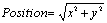But if an out of control condition is detected, the correction must be made with either the x and/or y adjustments.

### Capability Ratios

Capability ratios for true position are based on the probability of the position falling beyond the circular tolerance region. Therefore, the natural variability must also be expressed in terms of a circular region. Variability is measured in both the x and y planes. For the purpose of calculating capability ratios, the largest of the two sources is used (this is the most conservative approach). The ratios compare the area of the tolerance region to the area of the natural variability region (6 sigma spread) using the following formulas: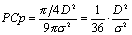Where PCp is the Positional Cp.Where PCpk is the Positional Cpk.

Using this approach, both the tolerance region and natural variability region are circular. In reality, however, the natural variability region is elliptical (x and y standard deviations are usually slightly different and directional). Enabling the confidence region from the Graph Setup (specifying a user-defined confidence level) one can see the elliptical nature of the true position data cloud.

Reference:

Dr. K. S. Krishnamoorthi. Capability Indices for Processes Subject to Unilateral and Positional Tolerances. Quality Engineering. 2(4). 461-471, 1990.

### Setting Up the Polar Plot

Using two linked test characteristics (usually x and y) create a Scatter Plot. From the Graph Setup, specify the positional tolerance; enable the tolerance region and natural variability region markers.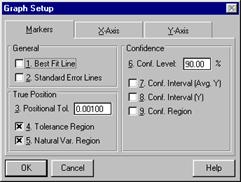Graph Setup dialog box

You will also need to specify specification limits for the x and y values. If x and y do not have tolerances, use the rule of thumb:

Positional Tolerance × .70711

Where .70711 is 45° sine

Example: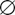.010 Tol. × .70711 = .0071 = ± .0035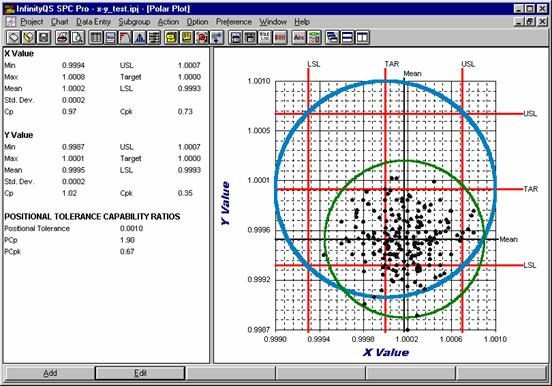Polar Plot showing true position values, the tolerance region and natural variability region.

### Setting Up the Control Charts

Nothing special here; you set up one control chart for the x values and another control chart for the y values. Optionally, a Group chart can be used to plot both x and y data streams on the same chart.

Need help?
​For further information contact GetInTouch@infinityqs.com
Toll Free: 1.800.772.7978​

### Take the first step from quality to excellence

Take the Next Steps